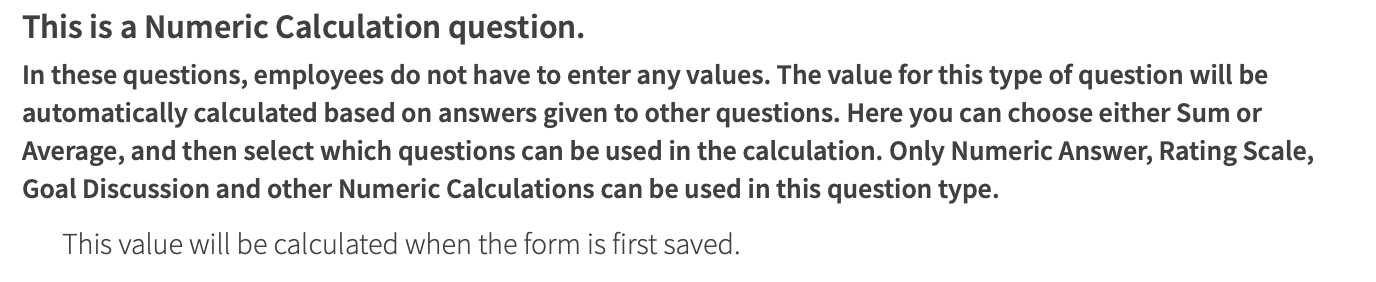# Introduction

PerformYard includes a number of different types of questions that you can add to your reviews. For each question type, you can add primary question text and then optional question subtext; some questions then have additional options or parameters required. For each question type shown below, you will see the input option(s) as well as the result as it appears in a review.

## Instructions Type Question

Instructions are actually not questions at all, but allow you to create sections and provide guidance to those employees completing a form.

### Setting up an Instructions Question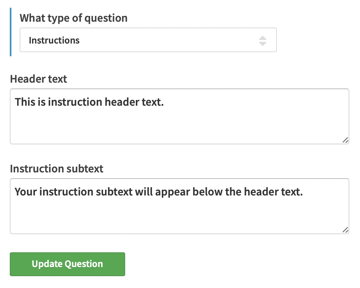### Instructions questions in practiceThe most open-ended question type, the long answer provides text boxes where employees can write detailed answers.

### Setting up a Long Answer Question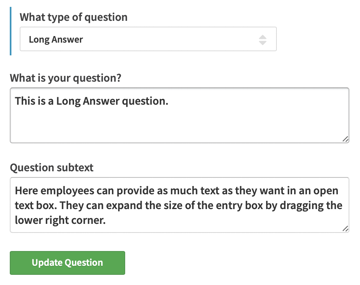### Long Answer questions in practice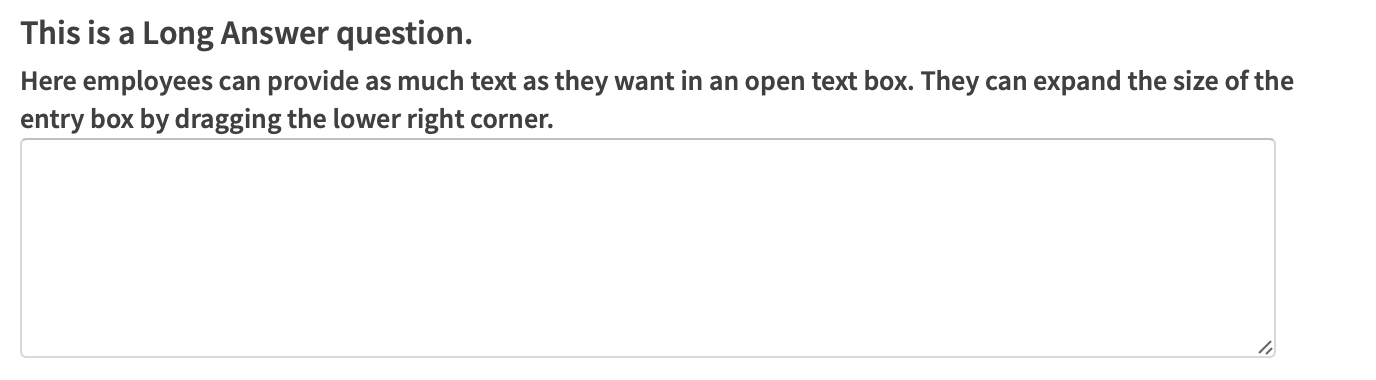## Multiple Line Answer Type Question

These are bulleted lists where employees can add as many or as few bullets as they want.

### Setting up a Multiple Line Question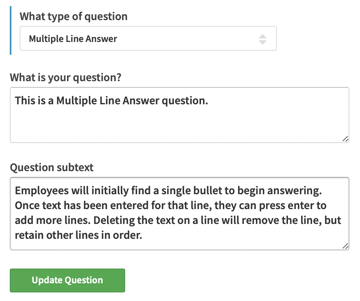### Multiple Line questions in practice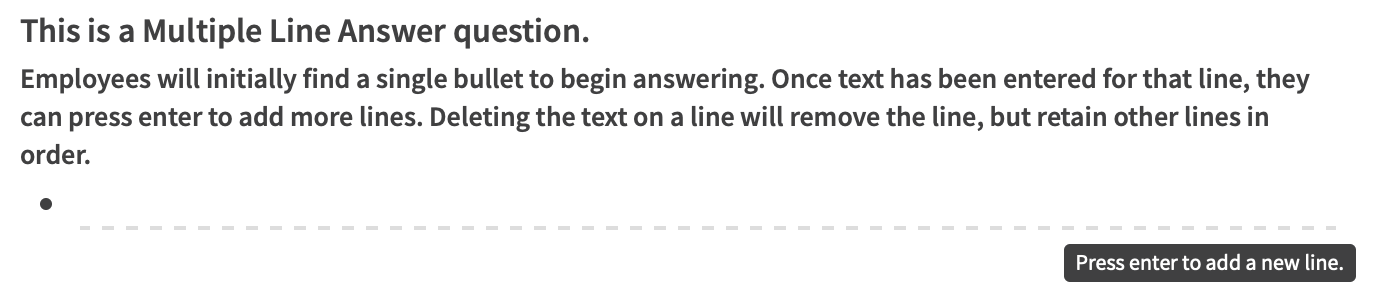## Numeric Type Question

These are questions seeking an answer in the form of a number only; includes options for min/max and to allow decimals/integers.

### Setting up a Numeric Question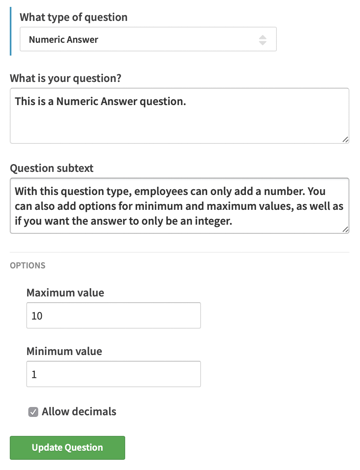### Numeric Questions in practice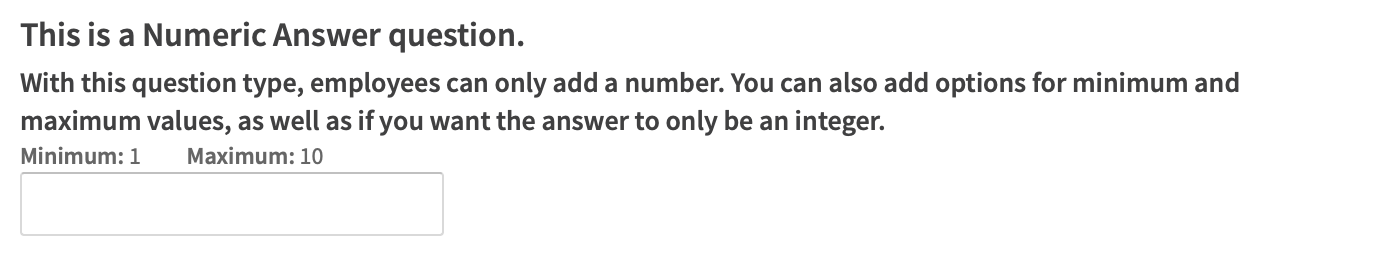## Date Type Question

For these questions, respondents select a date using a calendar function.

### Setting up a Date Question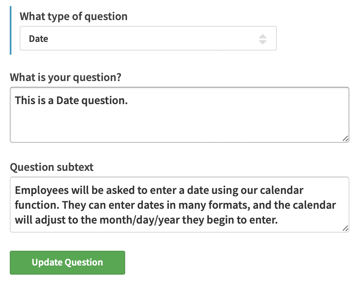### Date questions in practice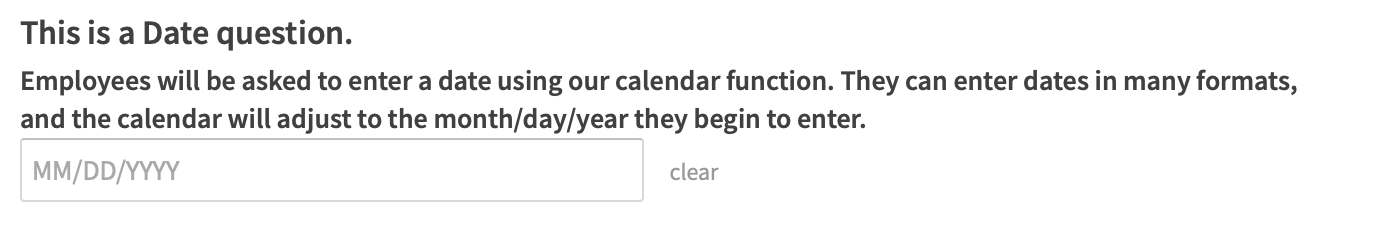## Multiple Choice Type Question

These are questions where employees can select from a pre-defined list of choices; you may include a maximum of 20 answer choices. The default for multiple choice questions is where an employee can select only one answer; to allow for employees to select more than one answer, check the box for Allow selection of multiple answers. Note: we recommend adding to the question subtext any specific instructions on how many answers to select.

### Setting up a Multiple Choice Question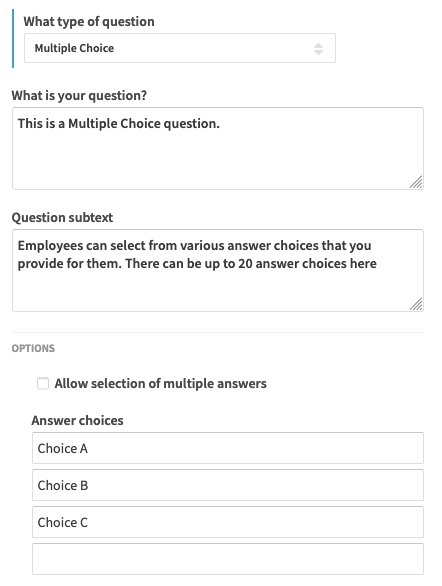### Multiple Choice questions in practice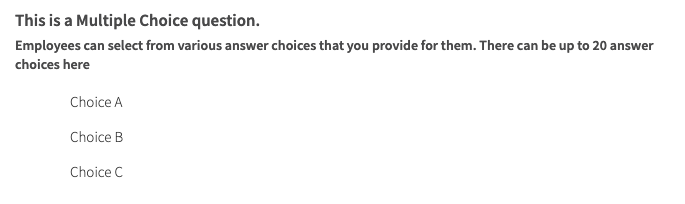## Rating Scale Type Question

These questions allow employees to select from a pre-defined list (like a multiple choice), but where each point on the scale has an associated numeric value such that it can be used in a calculation. Rating scale questions also include definitions for each point that appear on hover. Note that the associated numeric value is what will export in a review cycle CSV for data comparison purposes, while the selected words will appear in PDF exports. Also note that if the associated numeric value is blank, then the question will not factor into any numeric calculation if that rating choice is selected.

### Setting up a Rating Scale Question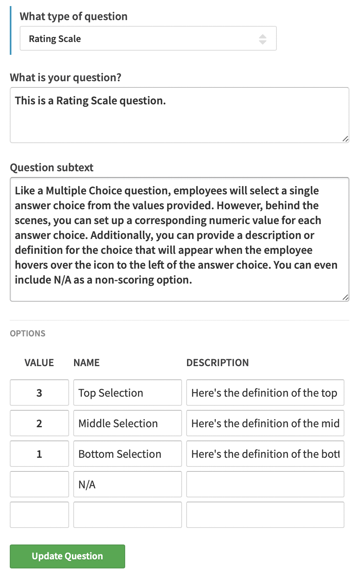### Rating Scale questions in practice

These questions provide the option to a single answer from a list of descriptions.  A numeric value can be associated with each option to allow for its use in calculations.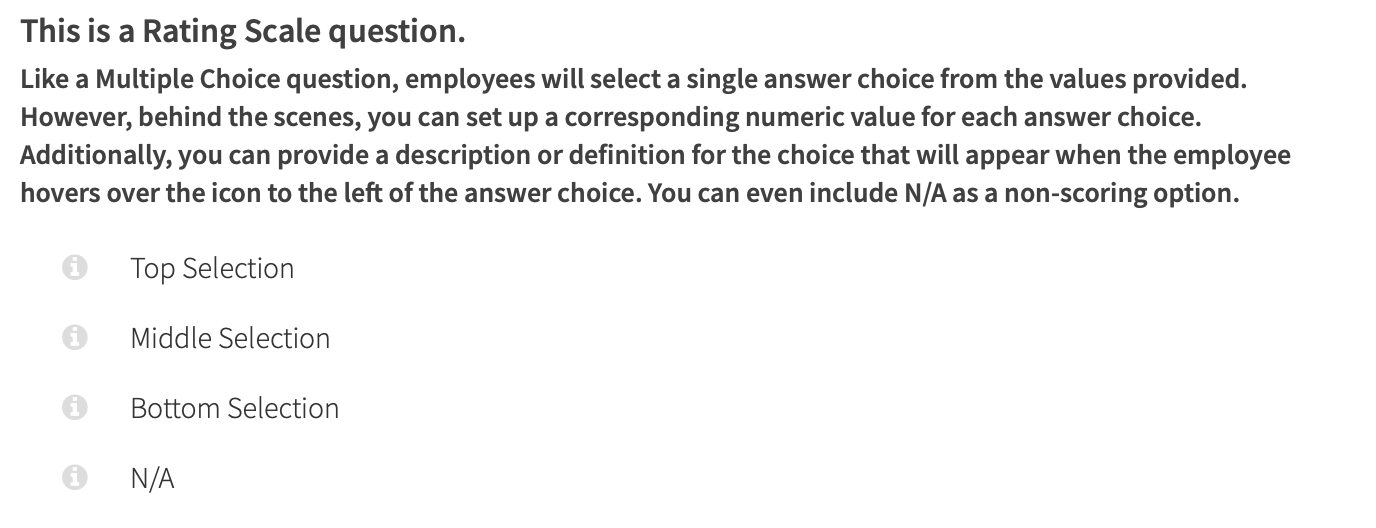Here's how the description text looks in practice on hover.## Goal Discussion Type Question

These questions provide the option for employees to select one or more of the review subject's goals and discuss their achievement; these may also include the option to score, rate, and/or weight performance on the goal for calculations.

### Setting up a Goal Discussion Question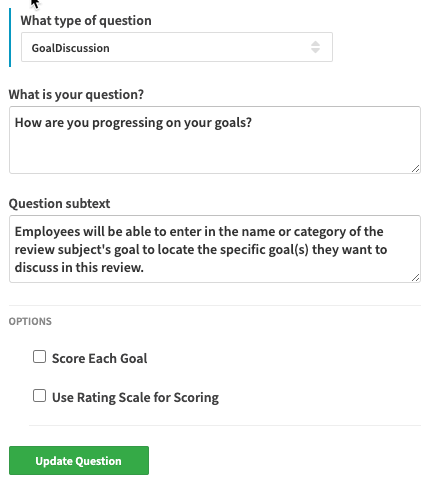#### Option to Score Each Goal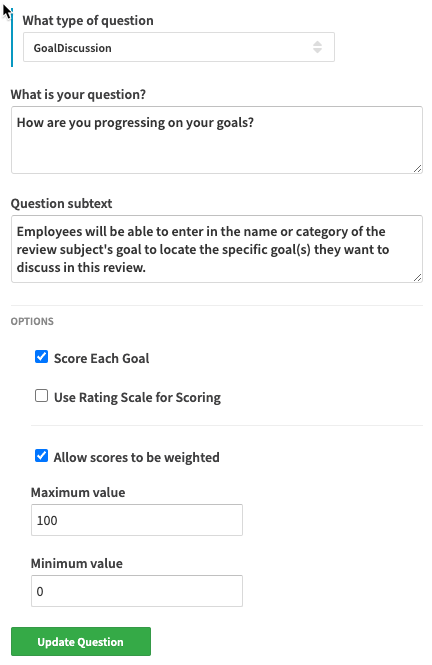#### Option to Score Each Goal Using a Rating Scale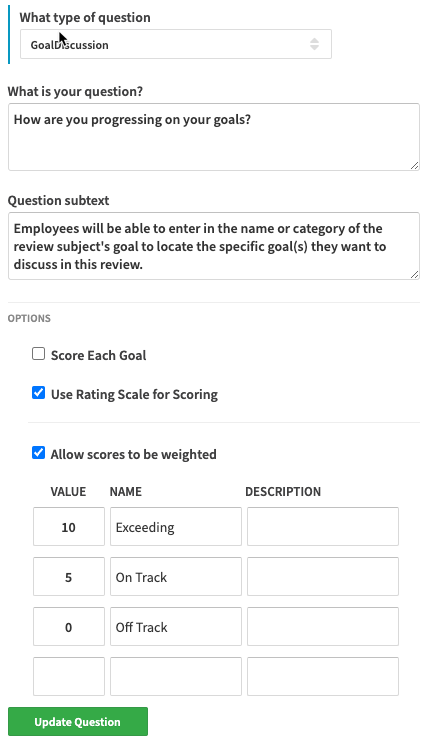Goal Discussion questions in practice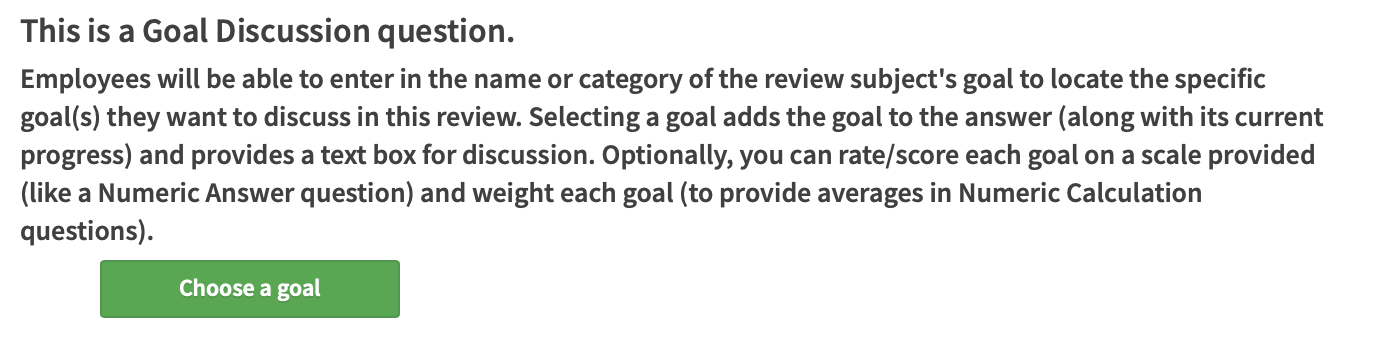## Goal Creation Type Question

These questions allow the employee to create new goals for the subject employees one and a time. Those new goals can then be worked on by the subject employee, but will always be tied to having been created in the review.

### Setting up a Goal Creation Question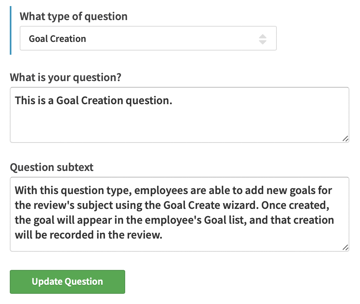### Goal Creation questions in practice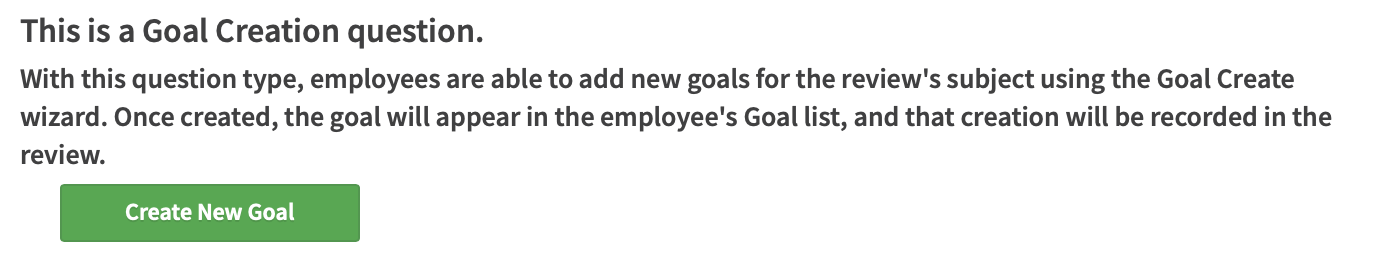## Numeric Calculation Type Question

As the name implies, these questions automatically calculate a value based on answers to other numeric questions, rating scale questions and rated goal discussion questions. You can also weight individual question contributions to the calculated value (defaulted to equal). A few important notes: first, the inclusion of a goal discussion question in a calculation will include all scored/weighted goals that are selected into that one factor of the overall calculation; second, skipping any question that is part of a numeric calculation will mean that question is not factored into the result and the weight of that question will be distributed to the other questions proportionately (i.e. a skipped question is ignored, not treated as a zero); third, any rating scale answer that is selected where the Value was left blank will be treated like a skipped question in the calculation.

Note there is also an option within Numeric Calculation to "Hide calculation on review form for all users" which means that the question & its answer will not be shown on screen when viewing the form by any user or be available in any PDF. The numeric calculation will only be available in CSV downloads or for use in cycle visualizations/performance graphs.

### Setting up a Numeric Calculation Question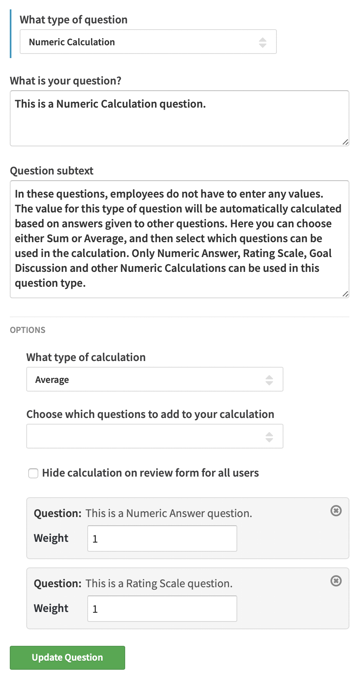### Numeric Calculation questions in practice